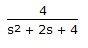Courses

# Test: Instrumentation & Process Control - 2

## 25 Questions MCQ Test Instrumentation and Process Control | Test: Instrumentation & Process Control - 2

Description
This mock test of Test: Instrumentation & Process Control - 2 for Electrical Engineering (EE) helps you for every Electrical Engineering (EE) entrance exam. This contains 25 Multiple Choice Questions for Electrical Engineering (EE) Test: Instrumentation & Process Control - 2 (mcq) to study with solutions a complete question bank. The solved questions answers in this Test: Instrumentation & Process Control - 2 quiz give you a good mix of easy questions and tough questions. Electrical Engineering (EE) students definitely take this Test: Instrumentation & Process Control - 2 exercise for a better result in the exam. You can find other Test: Instrumentation & Process Control - 2 extra questions, long questions & short questions for Electrical Engineering (EE) on EduRev as well by searching above.
QUESTION: 1

Solution:
QUESTION: 2

Solution:
QUESTION: 3

### The maximum service temperature for fibre glass used as thermocouple wire insulation material is __________ �C.

Solution:
QUESTION: 4

Which of the following controllers has the least maximum deviation ?

Solution:
QUESTION: 5

Volumetric expansion is the working principle of the __________ thermometers.

Solution:
QUESTION: 6

Which of the following measurements can be made by the measurement of emf ?

Solution:
QUESTION: 7

A magnetic flowmeter is

Solution:
QUESTION: 8

Zirconia probe is used for the continuous measurment of __________ flue gases going out of the furnace.

Solution:
QUESTION: 9

Strain gage pressure transducers are used to measure __________ pressures.

Solution:
QUESTION: 10

The transfer function for a PID controller is (where, τ i is the integral (reset) time and τD is the derivative time.)

Solution:
QUESTION: 11

Compositional analysis of __________ is done using mass spectrometer.

Solution:
QUESTION: 12

The function of a transducer is to

Solution:
QUESTION: 13

Which of the following thermocouples will give the highest output for the same value of hot and cold junction temperature ?

Solution:
QUESTION: 14

Nichol's chart deals with

Solution:
QUESTION: 15

A proportional controller with a gain of Kc is used to control a first order process. The offset will increase, if

Solution:
QUESTION: 16

The symbol for "capillary line" in instrumentation diagram is

Solution:
QUESTION: 17

__________ is undesirable in thermocouples used in industries,

Solution:
QUESTION: 18

For an input forcing function, X(t) = 2t2, the Laplace transform of this function is

Solution:
QUESTION: 19

Select the correct statement from the following.

Solution:
QUESTION: 20

Continuous shell temperature measurement in a liquid-liquid heat exchanger is done by a

Solution:
QUESTION: 21

The __________ of a vapor pressure thermometer is a secondary element.

Solution:
QUESTION: 22

Emf developed by a thermocouple, while measuring a temperature of 600�C is about 5.5 mV. The type of thermocouple used is

Solution:
QUESTION: 23

Which of the following relates the absorption & evolution of heat at the junctions of a thermocouple to the current flow in the circuit?

Solution:
QUESTION: 24

The second order system with the transfer functionhas a damping ratio of

Solution:
QUESTION: 25

In a shell and tube heat exchanger, the flow rate of heating/cooling fluid is the __________ variable.

Solution: